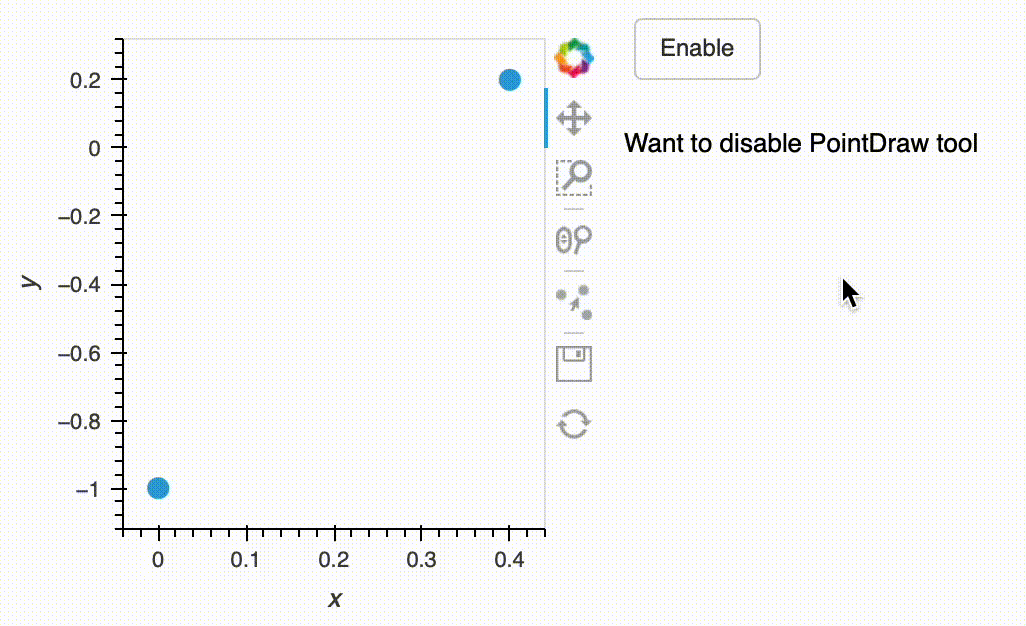# How to programmatically change active_tools of a bokeh plot?

Hi, is there a way to programmatically change which tools are active in a bokeh plot?For context, I am trying to incorporate the MeasurementTool (as suggested in ) with a fairly complex app based around PointDraw, and the problem is that need to disable the PointDraw tool when using the measurement tool, otherwise the clicks are also registered as adding of the points.

I guess an alternative would be to capture when the user toggles the tool on/off in the toolbar and use that to enable/disable the measurement tool, but that is less explicit (for the user)…

``````import holoviews as hv
import panel as pn
import param

class DummyClass(param.Parameterized):
points_stream = param.ClassSelector(class_=hv.streams.PointDraw, )
source_plot = param.ClassSelector(class_=hv.Points, )
enable = param.Boolean()

def __init__(self, data, **params):
super().__init__(**params)
self.source_plot = hv.Points(data=data, kdims=["x","y"]).opts(size=10)

self.points_stream = hv.streams.PointDraw(
data=self.source_plot.columns(),
source=self.source_plot,
empty_value='',
)

def show_mode(self):
if self.enable:
self.source_plot.opts(active_tools=['point_draw'])
return "PointDraw enable"
else:
self.source_plot.opts(active_tools=[])
return "PointDraw disabled"

pn.extension()
hv.extension("bokeh")
data = {"x":[0,.4],'y':[-1,.2]}
mt = DummyClass(data = data)
pn.Row(
mt.source_plot,
pn.Column(
pn.widgets.Toggle.from_param(mt.param.enable, width=20),
mt.show_mode
),
).servable()
``````

I’m on holoviews 1.15.4.